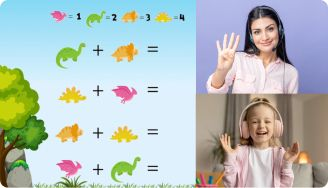# Operation With Fractions Worksheets

Fractions are a very interesting element of math. Put simply, a fraction is a number that represents a part of a whole number. It’s typically represented by a combination of two numbers arranged on top of each other. These numbers are separated by a horizontal dash and are called the numerator and denominator. To operate with fractions, you must first understand what they are. You’ll also need to understand the rules of different mathematical operations (e.g addition, subtraction, multiplication, etc). Having a firm grasp of the concept of fractions will help you work with them seamlessly. You probably already know this.

Leave your email and we will send you worksheets

worksheets sent successfully

However, for an average young learner, digesting all this information can be tricky. That’s why you need an operation with fractions worksheet.

## About the Operations with Fractions Worksheet

The fraction operations worksheet is a handy learning tool that covers the basics: from definition of fractions to numerous operations. The worksheets comprise of a wide range of exercises of varying difficulty levels.

1:1 Math Lessons

Want to raise a genius?
Start learning Math with BrighterlyThese exercises are carefully curated to bolster and test young learners’ knowledge of fractions. More importantly, kids are also equipped with logical and critical thinking skills. At the end of the day, you’ll have a mini problem solver on your hands.

## Why Do You Need Mixed Operations with Fractions Worksheet PDF?

Leave your email and we will send you worksheets

worksheets sent successfully### Operation With Fractions Worksheets PDF

View worksheet

Operation With Fractions Worksheet### Operation With Fractions Worksheets PDF

View worksheet

Fraction Operations Worksheet### Operation With Fractions Worksheets PDF

View worksheet

Operations With Fractions Worksheet### Operation With Fractions Worksheets PDF

View worksheet

Mixed Operations With Fractions Worksheet PDF

The operations with fractions worksheet pdf comes with several perks for young learners and parents alike. It strengthens children’s understanding of fractions and mathematical operations. The exercises are also designed to test their skills and fill in any learning gaps.

So, if you’re looking to teach your kid all about fractions, this worksheet is an excellent choice.

### More Fractions Worksheets

Book 1 to 1 Math Lesson• Specify your child’s math level
• Get practice worksheets for self-paced learning
• Your teacher sets up a personalized math learning plan for your childBook 1 to 1 Math Lesson